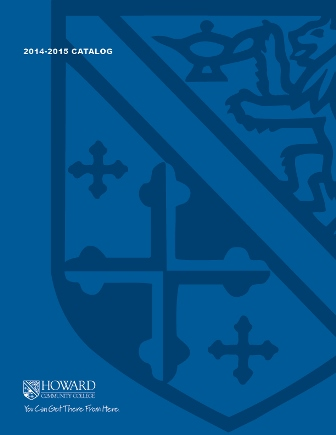2017-2018 College Catalog# MATH 037 Mathematical Foundations

This course is for students in developmental mathematics who plan on taking a Statistics or liberal arts mathematics course. Using data analysis, the course develops and integrates quantitative relationships, algebraic reasoning, functional reasoning, probabilistic and statistical reasoning with a focus on communication skills. Students will develop conceptual, procedural, and technological tools that support the use of key mathematical concepts in a variety of real-world contexts.

4

### Prerequisite

An appropriate score on the mathematics placement test, or a grade of B or higher in MATH 061, or a grade of C or higher in MATH 063 AND eligible to enroll in ENGL 086 or ENGL 096

FYEX 100

4 hours weekly

### Course Objectives

1. 1. Quantitative Relationships: Students will describe numerical relationships and solve real-world problems involving categorical and quantitative data.
2. 2. Patterning and Algebraic Reasoning: Students will transition from specific and numeric reasoning to general and abstract reasoning using the language and structure of algebra to investigate, represent, and solve problems.
3. 3. Functional Reasoning: Students will represent linear and exponential relationships using multiple representations.
4. 4. Probabilistic Reasoning: Students will investigate chance processes and develop, use, and evaluate probability models.
5. 5. Statistical Reasoning: Students will use random sampling to draw inferences about a population and develop understanding of statistical variability.
6. 6. Communicate mathematical concepts using appropriate symbols, notation, and vocabulary.
7. 7. Identify and implement appropriate technologies to complete tasks involving data analysis.

### Course Objectives

1. Quantitative Relationships: Students will describe numerical relationships and solve real-world problems involving categorical and quantitative data.

2. Patterning and Algebraic Reasoning: Students will transition from specific and numeric reasoning to general and abstract reasoning using the language and structure of algebra to investigate, represent, and solve problems.

3. Functional Reasoning: Students will represent linear and exponential relationships using multiple representations.

4. Probabilistic Reasoning: Students will investigate chance processes and develop, use, and evaluate probability models.

5. Statistical Reasoning: Students will use random sampling to draw inferences about a population and develop understanding of statistical variability.

6. Communicate mathematical concepts using appropriate symbols, notation, and vocabulary.

7. Identify and implement appropriate technologies to complete tasks involving data analysis.